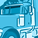# App Development

Announcements
QlikWorld online is next week! REGISTER NOW
cancel
Showing results for
Did you mean:Employee

## change line color by comparison target and actual value

I am trying to change line color of accumulated two measure's value comparison.

1.Target value : rangesum(above(sum([Target Value]),0,rowno()))

2.Actual Value: rangesum(above(sum([Actual Value]),0,rowno()))

I want to change the actual value line color as follows condition.

Target value > Actual value = line color is red and Target value < Actual value is green.

How can I change the color?Contributor

@tsitv wrote:

I am trying to change line color of accumulated two measure's value comparison.

1.Target value : rangesum(above(sum([Target Value]),0,rowno()))

2.Actual Value: rangesum(above(sum([Actual Value]),0,rowno()))

I want to change the actual value line color as follows condition.

Target value > Actual value = line color is red and Target value < Actual value is green.

How can I change the color?

Actually, I didn't understand your target value and actual value formulas. Please, can you elaborate more?

Regards, John